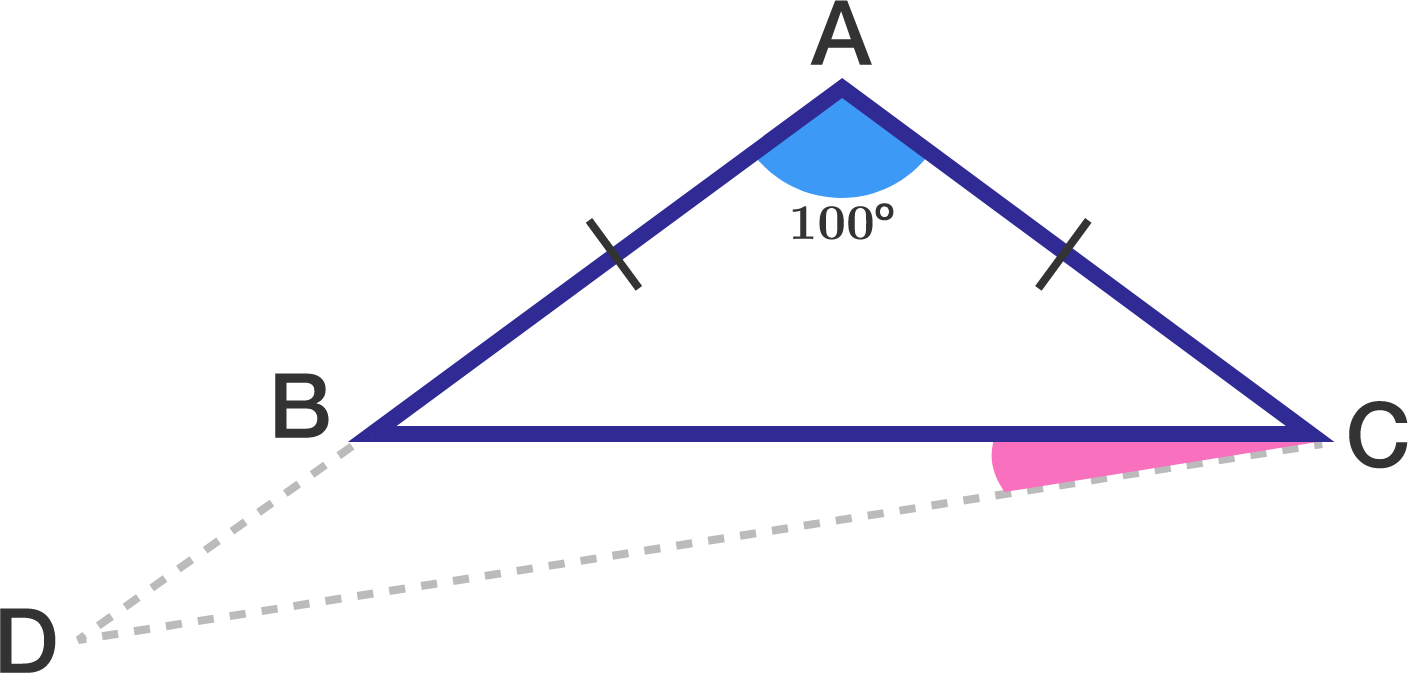# A geometry problem by Stalin Sahoo

Geometry Level 3In triangle $ABC,$ $AB = AC$ and $\angle BAC = 100^\circ.$ If $\overline{AB}$ is extended to $D$ such that $AD=BC,$ find $\angle BCD$ (in degrees).

×